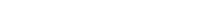# Math riddles Level 56 Answer with Hints and solutionsMath riddles game level 56 Answer with solution android game developed by Black games. Scroll Below to find details.

Math Riddles tests your IQ with mathematical puzzles. Challenge yourself with different levels of math puzzles and stretch the limits of your intelligence. Every IQ game is prepared with an approach of an IQ test. Improve your mathematics, challenge your brain with this game. There are many hard levels which you may not have solved then you can take help from here.

Math app level 56:

11,17=36

18,23=25

4,12=64

29,32=?Solution: square of the difference of given numbers

If there is any Doubt/discrepancy then please let me know in comments or you are unable to understand solution then I will surely help you.

### 3 thoughts on “Math Riddles App Level 56 Solution Android”

•December 9, 2019 at 5:02 pm

11,17=36,,,,,11-17 =(-6)²= 36

18,23=25,,,18-23= (-5)²= 25

4,12=64

29,32=?,,, (-3)²= 9

•December 12, 2019 at 10:33 pm
•